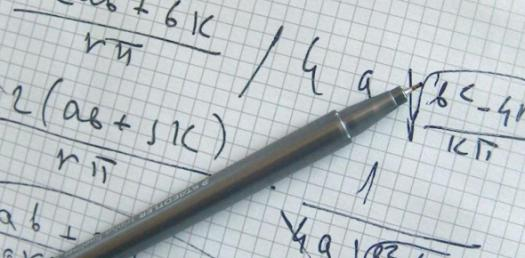# Mathematical Skills: Dimensional Analysis Quiz!

9 Questions | Total Attempts: 44Settings.

Related Topics
• 1.
16 fl oz/h is about ____ mL/min. Your answer should be between 5 and 15. Enter only the number, rounded to two decimal places.
• 2.
In meters per second, how fast is 1,550 feet per minute? Your answer should be between 5 and 15. Enter the number only, rounded to 2 decimal digits.
• 3.
A storage bin is being filled at a rate of 2,350 lb / h. What is the rate in kg/min? Your answer should be between 15 and 25 kg/min. Enter the number only, rounded to the nearest hundredth.
• 4.
A certain SUV can travel an average of 18 miles per gallon of gasoline.  How many kilometers can the SUV travel with one liter of gasoline? You answer should be between 5 and 15. Enter only the number, rounded to the nearest hundredth.
• 5.
When preparing to cook, Joanna can chop 2 pounds of carrots in 3 minutes. How many ounces can she chop per second? Your answer should be between 0 and 10. Enter only the number, rounded to the nearest hundredth.
• 6.
A vehicle can travel 11 kilometers per liter of gasoline. How many miles per gallon is this? Your answer should be between 20 and 30.  Enter only the number, rounded to the nearest hundredth.
• 7.
70 mph is approximately ______ feet / second. Your answers should be between 100 and 110. Enter only the number, rounded to the nearest hundredth.
• 8.
150 fl oz / day is about _________ L / h. Your answer should be between 0 and 10. Enter only the number, rounded to the nearest hundredth.
• 9.
20 oz / min is about ____ qt / day. Your answer should be an integer between 875 and 975. Enter only the number.